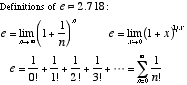index: click on a letter A B C D E F G H I J K L M N O P Q R S T U V W X Y Z A to Z index index: subject areas numbers & symbols sets, logic, proofs geometry algebra trigonometry advanced algebra & pre-calculus calculus advanced topics probability & statistics real world applications multimedia entrieswww.mathwords.com about mathwords website feedback

 e e ≈ 2.7182818284.... is a transcendental number commonly encountered when working with exponential models (growth, decay,and logistic models, and continuously compounded interest, for example) and exponential functions. e is also the base of the natural logarithm.See also Euler's formula eiπ + 1 = 0